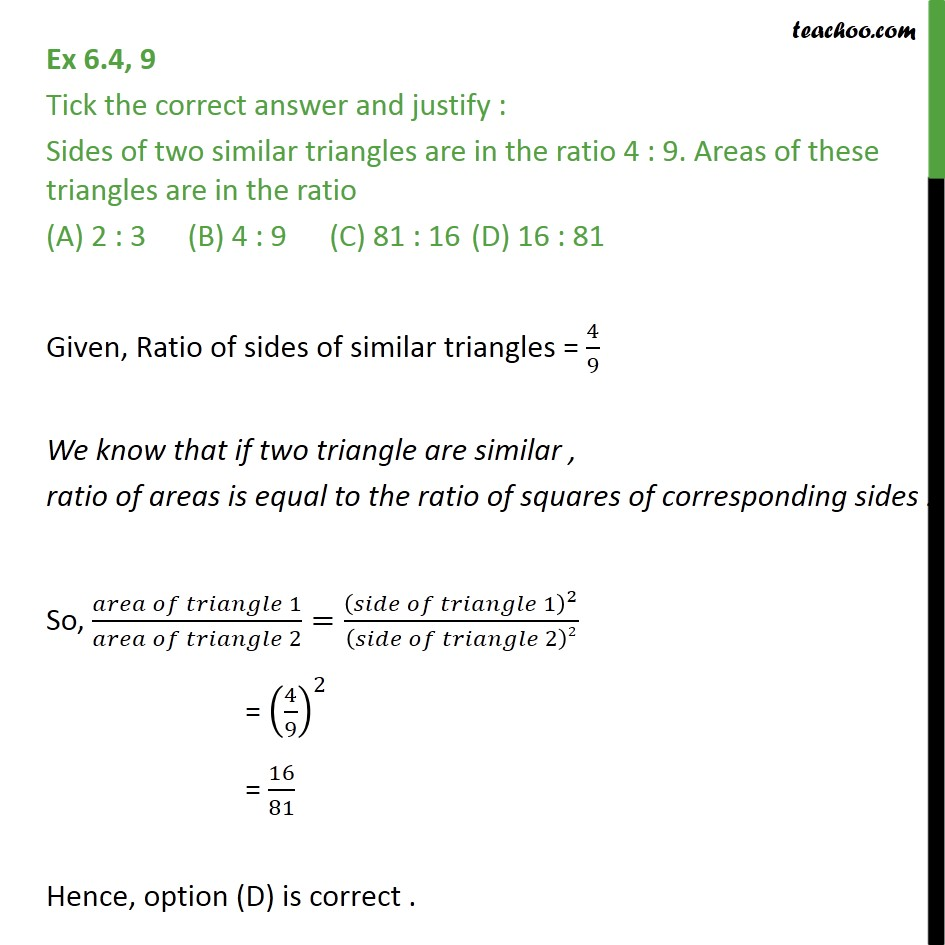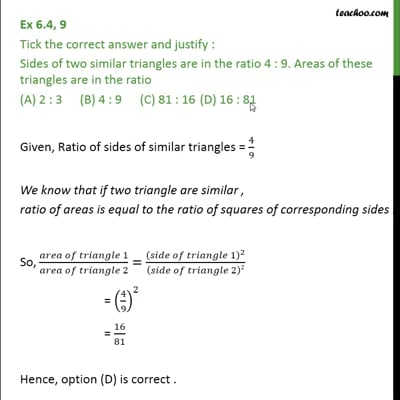Area of similar triangles

Chapter 6 Class 10 Triangles
Concept wiseThis video is only available for Teachoo black users

Solve all your doubts with Teachoo Black (new monthly pack available now!)

### Transcript

Ex 6.4, 9 Tick the correct answer and justify : Sides of two similar triangles are in the ratio 4 : 9. Areas of these triangles are in the ratio (A) 2 : 3 (B) 4 : 9 (C) 81 : 16 (D) 16 : 81 Given, Ratio of sides of similar triangles = 4/9 We know that if two triangle are similar , ratio of areas is equal to the ratio of squares of corresponding sides . So, ( 1)/( 2)=( 1)^2/( 2)2 = (4/9)^2 = 16/81 Hence, option (D) is correct